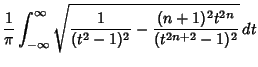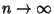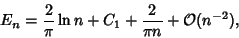## Kac Formula

The expected number of Real zerosof a Random Polynomial of degreeis(1)(2)

As,(3)

where(4)

The initial term was derived by Kac (1943).

References

Edelman, A. and Kostlan, E. How Many Zeros of a Random Polynomial are Real?'' Bull. Amer. Math. Soc. 32, 1-37, 1995.

Kac, M. On the Average Number of Real Roots of a Random Algebraic Equation.'' Bull. Amer. Math. Soc. 49, 314-320, 1943.

Kac, M. A Correction to `On the Average Number of Real Roots of a Random Algebraic Equation'.'' Bull. Amer. Math. Soc. 49, 938, 1943.